Home > A2C > Chapter 7 > Lesson 7.2.3 > Problem7-129

7-129.
1. Write three different, but equivalent, expressions for each of the following logs. For example: log(73/2) can be written as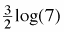,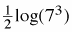,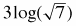, etc. Homework Help ✎

1. log(82/3)

2. −2log(5)

3. log(na)bo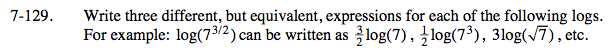Use the properties of logarithms and exponents to help you rewrite these.
Simplify different parts of the expression to create new, equivalent expressions.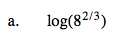Simplify the argument:

Simplify the argument further:

$\text{The Power Property of Logarithms is log}_m(a^n)=n\cdot \log m(a), \text{ so one way to rewrite this expression is }\frac{2}{3}\log(8).$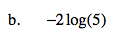Use the Power Property of Logarithms in reverse here.
Since n · logm(a) = logm(an), −2 log(5) = log(5−2).

Find two different ways to rewrite 5−2
(there are more than two ways to do this).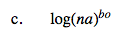Refer to parts (a) and (b).